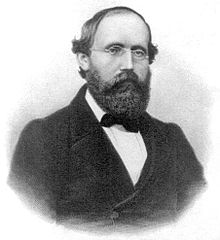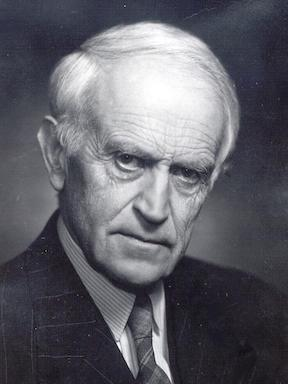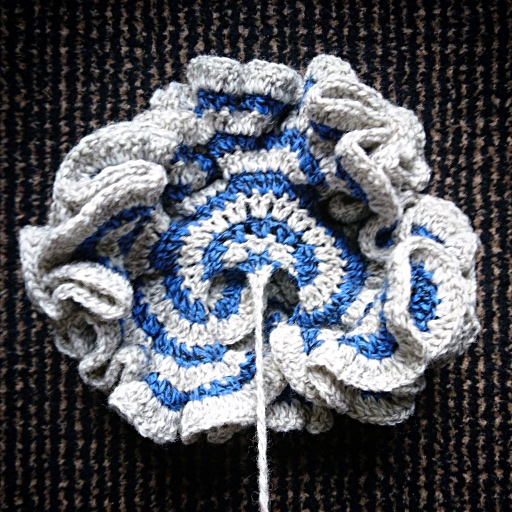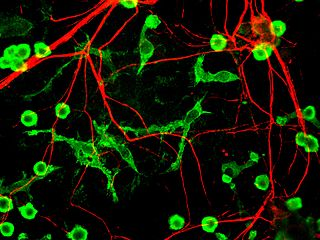Johannes Vermeer: Girl with a pearl earring

# Forum for matematiske perler(og kuriositeter)

Om Forum for matematiske perler (og kuriositeter)
2001/2002 · 2002/2003 · 2003/2004 · 2004/2005 · 2005/2006 · 2006/2007 · 2007/2008 · 2008/2009 · 2009/2010 · 2010/2011 · 2011/2012 · 2012/2013 · 2013/2014 · 2014/2015 · 2015/2016 · 2016/2017 · 2017/2018 · 2018/2019 · 2019/2020 · 2020/2021 · 2021/2022 · 2022→.

## Foredrag 2018–2019

### Yuri Matiyasevich: Hilberts Tenth Problem and some other difficult problems🔗

Sted:
Lunsjrommet i 13. etg., Sentralbygg 2
Tid:
–13:00 (merk ukedagen)
Slides:
Available from the speaker's own website

The undecidability of Hilbert's tenth problem (H10) about Diophantine equations turned out to be a powerful tool for establishing the undecidability of many other decision problems. The technique developed for proving the undecidability of H10 gave the possibility to find unexpected relationships between Diophantine equations and some other classical mathematical problems.

Yuri Matiyasevich (Ю́рий Влади́мирович Матиясе́вич) is famous for his solution of Hilberts Tenth Problem: “Does there exist an algorithm whereby one can solve in a finite number of operations any Diophantine equation?” In 1970, when he was 22 years old, Matiyasevich gave a negative answer to Hilberts problem.

### Peter Lindqvist: The Riemann Hypothesis🔗Sted:
Lunsjrommet i 13. etg., Sentralbygg 2
Tid:
–13:00
Ark fra dokumentkameraet:
2019-04-05_riemann.pdf

The Riemann Hypothesis is one of the great problems in mathematics. No valid proof (or disproof) is known. It states that all complex zeros of the zeta function $\zeta(s)$ are on the critical line $s = \tfrac{1}{2}+ it,\,-\infty < t < \infty.$ My talk will be an attempt to explain the influence of the location of the zeros on the distribution of the prime numbers. (There is no elementary explanation to offer!)

In his memoir Ueber die Anzahl der Primzahlen unter einer gegebenen Grösse Bernhard Riemann gave in 1859 an explicit formula for the number of primes less than a given bound. The formula runs over all the zeros of the zeta function. The Riemann Hypothesis is equivalent to an absolute error term of the order $O(\sqrt{N}\log(N))$ in the Prime Number Theorem. (Without any hypothesis, no error term even of the form $O(N^{1-\varepsilon})$ has been proved.) Under the Hypothesis one has $$\Bigl|\pi(N)-\int_{0}^{N}\frac{dy}{\log(y)}\Bigr| <\,\frac{1}{8\pi}\sqrt{N}\log(N),\quad N \geq 2657$$ (von Koch 1901, Schoenfeld 1976), where $8\pi$ is an artefact and $\pi(N) =$ the number of primes $\leq N$.

Hardy proved that infinitely many zeros lie on the critical line, Selberg generalized it to a positive portion of all zeros. Levinson (1974) extended it to include 33% of the zeros. Conrey (1989) placed 40% of them on the critical line. “Vita brevis, ars longa, occasio …”.

\begin{aligned} \pi(x) &= \Pi(x)-\tfrac{1}{2}\Pi(x^{1/2}) -\tfrac{1}{3}\Pi(x^{1/3}) -\tfrac{1}{5}\Pi(x^{1/5}) +\tfrac{1}{6}\Pi(x^{1/6})\cdots\\ \Pi_0(x) &= \int_o^x\frac{dy}{\log(y)} -\sum_k\mathrm{Li}(x^{\rho_k}) -\log(2) + \int_x^{\infty}\!\!\frac{dy}{y(y^2-1)\log(y)}\\ \zeta(\rho_k)&=0, \quad \rho_k =\beta_k +i\gamma_k, \quad x^{\rho_k } = x^{\beta_k}e^{i\gamma_k\log(x)}\\ \Pi(x) &=\tfrac{1}{2}\bigl( \Pi_0(x+0) + \Pi_0(x-0)\bigr). \end{aligned}

### Celine Marie Løken Cunen: Statistical Sightings of Better Angels🔗Sted:
Lunsjrommet i 13. etg., Sentralbygg 2
Tid:
–13:00
Slides:
Statistical sightings of better angels

After the Second World War, humanity experienced fewer large wars than in any other period in history. This empirical pattern has become known as the Long Peace among peace and conflict researchers, and it has generated a great number of explanations and discussion. Many of the discussions concern a question which is mostly statistical in nature: how special is really the Long Peace? Is the pattern simply a result of random fluctuations in the war-generating mechanism, or does it represent a significant change? In order to study these questions we have made use of various statistical tools: models for power-law distributed data, homogeneity tests, and methods for change-point detection.

This talk is based on joint work with Håvard Nygård and Nils Lid Hjort.

### Hans Munthe-Kaas, UiB: Symmetry: From Conway’s Magic Theorem to Archimedes’ Labyrinth and beyond🔗Sted:
Lunsjrommet i 13. etg., Sentralbygg 2
Tid:
–13:00
Slides:
Symmetry: From Conway’s Magic Theorem to Archimedes’ Labyrinth and beyond

At the University of Bergen, I have for the last three years held weekly ‘Mattesirkel’-lectures for especially motivated high school students. The topic ‘Symmetry and Group Theory' is inspiring for both the students and the lecturer. The classification of discrete symmetries of surfaces is a celebrated topic, but the classical proof of the 17 wallpaper groups is unfortunately beyond what can reasonably be presented to high school students. Recently John H. Conway introduced a ‘Magic formula’, based on topology, which fits well within the scope of Mattesirkelen. This formula can be used to classify discrete symmetries for spherical, plane and hyperbolic surfaces and yields the 17 wallpaper groups, the 7 frieze patterns and all 14 families of discrete spherical symmetries as special cases.

A year ago, the leader of the Botanical Garden in Bergen asked me to design a hedge-labyrinth as a new attraction. Inspired by ‘Mattesirkelen’, I ended up with a highly symmetric design. Under some reasonable assumptions, only one of the 17 wallpaper groups is suitable. The resulting labyrinth, called ‘Archimedes’ labyrint’, consists of 1234 yews (barlind) in 2m height and covers an area of about 800 m2. It opened in September 2018 and was presented in Science Magazine, October 2018

If time allows, I might mention briefly some of my own research on multivariate Chebyshev polynomials related to kaleidoscopic groups.

### Christian Skau: A century of Brun's sieve – a momentous event in prime number history🔗Sted:
Lunsjrommet i 13. etg., Sentralbygg 2
Tid:
–13:00
Slides:
Brun's sieve

In 1919 – a century ago – Viggo Brun succeeded in constructing a sieve that opened up new avenues to make progress on some famous conjectures about prime numbers. These conjectures, e.g., the Goldbach Conjecture and the Twin Prime Conjecture, were considered by the experts at the time to be unattainable. Remarkably, Brun started with the Eratosthenes' sieve from antiquity, and by elementary methods, using the so-called inclusion–exclusion principle, he was able to modify that sieve so that he could obtain highly non-trivial results. We will try to explain Bruns reasoning that led to his sieve.

(Incidentally, Viggo Brun was professor at NTH from 1923 to 1946.)

### Petter Bergh: The classification of finite simple groups🔗Adapted from an image by Dr Shwarz.

Sted:
Lunsjrommet i 13. etg., Sentralbygg 2
Tid:
–13:00

Mathematicians like to bring order to chaos, for example by classifying the structures we invent/discover. In abstract algebra, the fundamental kind of structure is the group: a set with one operation satisfying a few natural conditions. Groups show up everywhere in mathematics, often as sets encoding symmetries. For example, the groups that we now call Lie groups were introduced by Sophus Lie in connection with his work on differential equations.

Can we classify all groups in a meaningful way? This is hopeless unless we restrict ourselves to finite groups, that is, groups containing only finitely many elements. But even here the task is generally considered to be impossible. However, finite groups are built out of building blocks, namely finite simple groups, like integers are built out of prime numbers. The finite simple groups have been completely classified, in an effort that has involved lots of mathematicians and many thousand pages over fifty years. In this talk, we take a look at the history of this classification theorem.

### Markus Faulhuber: The ubiquitous constant and its siblings🔗

Sted:
Lunsjrommet i 13. etg., Sentralbygg 2
Tid:
–13:00
Slides:
2018-10-19_ubiquitous.pdf

At several points in mathematical literature, a constant called the ubiquitous constant with approximate value 0.847213 appears. In this talk, we are going to investigate some siblings of the ubiquitous constant, arising from theories going back to Gauss and, later on, Ramanujan.

Most probably, the constants appear at far more places in mathematics (and maybe even other sciences) than covered by this talk; nonetheless, we will try to discuss some of the following topics.

We will start with an engineering problem about optimal sampling strategies for Gaussian Gabor systems, the Strohmer and Beaver conjecture. This is, to the speaker's knowledge, the most recent example of the appearance of the siblings.

In addition, we will study an old unsolved problem from geometric function theory, concerning the true value of Landau's “Weltkonstante”. Further topics may include the temperature distribution on the 2-dimensional torus with varying metric, a coloring problem for lattices, a generalized arithmetic-geometric-mean method, Gauss' hypergeometric function 2F1 and complete elliptic integrals.

Despite the heavy nature of the topics, this is going to be a fun talk where we will just scratch the surface of each topic a bit.

### Kathrine Frey Frøslie: Statistics as a foreign language🔗Sted:
Lunsjrommet i 13. etg., Sentralbygg 2
Tid:
–13:00

Communication in medical research is storytelling by statistics. As a biostatistician at Oslo University Hospital, my job is to cooperate with clinicians to translate stories about clinically interesting blood, sweat and tears into numbers. When they tell their stories, clinicians must use methods and a language originating in a foreign culture: the statistical culture. It takes knowledge, practice and courage to succeed at a top level internationally, in disciplines which communicate differently from your mother tongue.

An important task of the statistical community is to approach researchers with other professional background and training, to achieve the best communication and consequently, the best results. “The best results” in interdisciplinary communication and advising comprise more than choosing the best statistical methods for a problem at hand. Statisticians should meet non-statisticians half-way and enhance their understanding and confidence in the foreign language of statistics. Advising, teaching, articles, books, popular science, TV-shows, TED-talks, apps, blogging, knitting – no means should remain unused. And to find the best channels, we must increase our awareness of our own superpowers.

Never stop having fun!

### Heidi Strømskag: Why do some people have problems comprehending mathematics?🔗Image credit: EnCor Biotechnology Inc.

Sted:
Lunsjrommet i 13. etg., Sentralbygg 2
Tid:
–13:00
Slides:
2018-09-07_comprehending.pdf

There are different descriptions of mathematical knowledge. Two quite opposite characterizations are given by two English philosophers and scientists, Roger Bacon (c. 1214–1294) and Bertrand Russell (1872–1970):

“The knowledge of mathematical things is almost innate in us … this is the easiest of sciences, a fact which is obvious in that no one's brain rejects it; for layman and people who are utterly illiterate know how to count and reckon.”

R. Bacon (cited in Dehaene, 2011, p. 260)

“Mathematics may be defined as the subject in which we never know what we are talking about, nor whether what we are saying is true.”

B. Russell (1918, p. 75)

How is it possible simultaneously to view mathematics as a system of transparent truths and a tangle of relations among opaque entities? Why is mathematics both so easy and so hard? The lecture will draw on recent research on neuroscience – it will give a brief overview of possible sources of learning disability in mathematics, and an explanation of how the brain works when we study and learn mathematics. This takes us to a semiotic perspective on mathematical activity – the impact of representations of mathematical object and transformations between them. Finally, we will see how this might be implemented in mathematics instruction.